# Convert a text value into a date in Excel

Sometimes you'll find yourself working with dates in an Excel spreadsheet that have been pasted or imported into Excel from another datasource. When that happens, Excel can treat those dates as text - in other words, they look like dates but don't behave like dates. For example you can't sort by date properly. This lesson looks at several ways you can convert a date which Excel is treating as text into a proper date value in Excel.

## Use the DATEVALUE function to convert text to a date.

The DATEVALUE function takes a text value and tries to convert it into a date. Whether it succeeds will depend on the text value you are trying to convert.

Date problems often occur when you import data from a CSV file (which is a text file format) and Excel doesn't recognise the dates in the file so it imports them as text instead. Another scenario is when you copy and paste date values into Excel from another file format.

Two tell-tale signs that you have a date problem are as follows.

• First, some or all of the dates will be left-aligned in the cell. Date values are normally right-aligned.
• Second, you'll notice when you click on a cell containing a date that it contains a value such as '2011/05/08. That apostrophe before the date is used to indicates to Excel that the value in the cell is text value, regardless of what it looks like. This feature is sometimes useful, but not in the situation we're looking at here.

The quickest way to solve the problem, is to use the DATEVALUE function as follows:

• You have the following scenario:• In a cell next to the cell you want to convert, enter this formula:• Note how the formula returns a number, 39092. This is the correct answer, but it has the wrong format.
• Excel treats all dates as serial numbers. It then uses formatting to present the serial number as a date.
• Normally, when you enter a date Excel recognises it as a date and formats it automatically. However, Excel actually stores the serial number the date represents.
• Clearly, this didn't happen in this example, so you'll have to format it manually.
• There are a number of ways to format a number as a date.
• The method is to use the Number formatting dropdown list on the Home ribbon bar. You can choose from Short Date or Long Date, or you can choose the More Number Formats option and either choose a different date format or create a custom date format. We'll cover custom date formats in another lesson.
•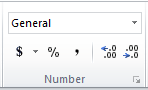• If you're using a Mac, the the only opNumber formatting dropdown only includes Date. To choose another date format, choose Custom...
• Once you choose a date format, the date should be presented as expected. This example shows the number in our example formatted as a date: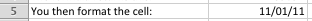• And that's the result we needed to get to - a text value that looked like a date but didn't behave like one, converted into the correct date format.

Of course, going to all of this effort doesn't make sense when you only have a few dates that haven't converted properly. However, if you have a large spreadsheet (e.g. with hundreds of values) the DATEVALUE function can be a real time saver.

## What happens when DATEVALUE doesn't work?

Sometimes, DATEVALUE just can't figure out how to convert the text value into a date. Usually it's because the text entered in the cell doesn't look like a date. Or, it could be that the text in the cell is actually a number that you know to be a date, but which Excel doesn't recognise as a date (or at least, doesn't recognise it for the date we know it to be).

• Here's an example of a text value that looks like a date but which just doesn't convert with DATEVALUE:
• 20120111
• It's not obvious to Excel that this is a date, so DATEVALUE doesn't know what to do with it - it will just return a #VALUE error instead.

## Option One - Combine DATEVALUE with the TEXT function

However, in this example we know that this is a date. We can break it out into its constituent parts - the year is 2012, the month is January, and the day is 11. Given this, we can use the TEXT function to help us out.

• The TEXT function converts a value into text. I know that sounds like the opposite of what what we're trying to do - but this method actually works very well.
• The syntax of the TEXT function is this: =TEXT(value, format_text)
• value is what we want to convert (or a cell containing that value)
• format_text is the format it should be converted to.
• In the example below, we're using a number that has been imported as a text value. Note that the TEXT function would also work if this had been imported as a number rather than as a text value: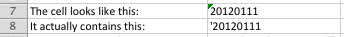• This is what the TEXT function does to the text value in B7:• We're nearly there! The only problem we have now is that while the date looks right, it is now a text value.
• You could solve this by combining the TEXT function and the DATEVALUE functions together: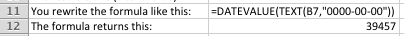• The final step would then be to format the cell in B12 as a date, as we did earlier.

## Option Two - A clever and obscure use of the TEXT function

Assuming the approach shown in Option One works in your particular example, Option Two will give you a better way of getting to the same answer.

• As before, we're trying to convert this value to a date:• This time, we'll only use the TEXT function to get the answer we want: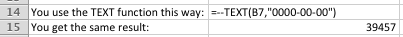• As above, you can now format this number as a date.
• You may well be trying to figure out how this formula manages to get the same result. Here's how:
• When we used the two minus signs (-) in the formula, we forced Excel to use the output of the the TEXT function in a calculation.
• To achieve this, Excel converted the text value into a number so it could complete the calculation without generating an error.
• What we actually did was convert the text value that the TEXT function generated into a negative number, and then back into a positive number.
• As you can see, this method is a quick and easy way to get the result we wanted, and achieves the same outcome without needing the DATEVALUE function.

## Option Three - Splitting the text into its constituent parts and recombining them into a date

The final method you can use when DATEVALUE doesn't deliver what you want is also the most cumbersome - but potentially the most flexible. Once you know this method, there are all sorts of uses you can put it to that have nothing to do with dates. I've already covered it in another lesson, Extract text from a cell in Excel, but it's worth showing you how to apply the method in this specific scenario.

• As before, we're trying to convert this text value to a date:• You can then use the LEFT, MID and RIGHT functions to extract the year, the month and the day from the text value. From there, you can use the DATE function to recombine them into something that Excel recognises as a date.
• The LEFT function allows you to choose a specified number of characters from the left end (i.e. the beginning) of a text value.
• The RIGHT function does the same as LEFT but starts from the right end of the text value.
• The MID function allows you to choose a specified number of characters from a specified starting point within the cell. In fact, you can do what we need with just the MID function, but let's look at how we might use all three.
• The DATE function takes a Year, a Month and a Day and combines them into a date (which Excel automatically formats as a date).
• Here's what this method looks like when applied to our problem: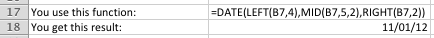• Let's break it out so you can see how it works:
• The LEFT function extracted the first four characters (the Year) from the cell B7 (i.e. the first four characters in the cell)
• The MID function extracted two characters (the Month) from B7 starting from the 5th character from the left
• The RIGHT function extracted two characthers (the Day) from B7 starting from the right (i.e. the last two characters in the cell)
• The DATE function combined the Year, Month and Day into a date, and then Excel formatted it for us.
• Some points to consider with this solution:
• You could have used the MID function in place of the LEFT and RIGHT functions had you wanted to. I leave that to you to figure out. I used all three functions in this example because the LEFT and RIGHT functions are sometimes very useful on their own, so I wanted you to see them in action.
• If you have a value that includes a time as well as a date, you can use the TIME function to convert the time portion of the text into a valid time, and add the results together to get a valid date/time. There is an example of converting text to both a date and time in the comments below.
• There are many, many scenarios where a formula that combines LEFT, RIGHT and MID will prove very handy. This has been just one of them.

## A final word on working with Dates in Excel

Dates are always problematic, and Excel's treatment of them can often cause confusion. One thing I have not addressed in this lesson until now is the fact that the US uses a date format that is different to most of the rest of the world. Specifically:

• The US refers to dates in the format "month, day, year"
• The rest of the world (ROW) refers to dates in the format "day, month, year"

That means that when you're working with dates in Excel, you need to know which date format your computer is set up to use (Excel takes its lead from the computer's date settings). Otherwise you can get some strange behaviour - and errors - showing up in Excel.

This example shows how confusion can arise.

• The date 04/05/2013 in the US refers to the 5th of April, 2013.
• In the ROW, it refers to the 4th of May, 2013.
• When sharing a spreadsheet with a colleage that uses a different date format to you, Excel will automatically switch the dates around so they appear in the correct format. But if you're working with a system that, unbeknown to you, is using a different format, you can get into all sorts of trouble.

This example shows how things can get even worse:

• The date 04/14/2013 in the US refers to the 14th of April, 2013.
• For the ROW, typing this into Excel would generate an error since there are only 12 months in the year, not 14.

On a day to day basis, most Excel power users are used to this problem and normally see it coming. But even the best of us can have a moment of confusion when a formula we tried to write comes back with the wrong answer or an error, before we realise what the cause is, and move on. In practical terms, when using the methods shown in this lesson for converting text values into dates, be aware that how you construct your formulas may depend on the date format being used in your copy of Excel.

## Our Comment Policy.

Add a comment to this lesson

### amazing! solved my problem

amazing! solved my problem which i was battling for 2 days in office.

### datevalue problem

hi there,

Very interesting solutions and it worked for one of my sheets. However, excel still returns the #VALUE! error message when I try to datevalue a date... Any other comments than the ones listed above?

Cheers,
Christian

### datevalue problem

date looks somethink like "21-12-2011 10:45" I have the feeling that somthing goes wrong due to the hour and minute after the date. Any idea how to get rid of the time?

### Date Format

Hi Chris

The DATEVALUE function is supposed to ignore time values. You'll find that removing the time value from your example doesn't solve the problem.

Question - can you confirm whether the quote marks are part of the value of the cell?

If they are, then they appear to be breaking the DATEVALUE function. I'm not sure why.

One solution you might consider is selecting just the date cells in your spreadsheet and doing a Find and Replace for the " character. By selecting only the cells you want, the Find/Replace will only operate on those cells. Removing the " character will convert the value into a date and you won't need a formula to help you.

However, I also found that this formula would do the trick, assuming that you've got the quote symbol (") at the start and end of the date values you're trying to convert:

=DATEVALUE(MID(A1,2,LEN(A1)-2))

This example assumes that the value I'm trying to convert to a date is in cell A1.

Essentially, the MID function takes the characters out from between the " characters, leaving you with just the raw date. The result is still considered by Excel to be a text value, though, so you need the DATEVALUE function as well.

David

### Same problem

Chris,

I found that I used the text function mentioned above and did ok with this same formatting (database output) I used =--TEXT(LEFT(datecell, 5),"00000000") Excel then took care of it after that for me.

Hope this helps and I'm not being redundant.

Dan

### Hi,

Hi,
U can use the INT(Cell No)....as =INT(the cell u want to convert to date only)

### EXCELL PROBLEM

HI I WANT TO KNOW HOW THIS FORMAT EX.13.10.2003 IS CONVERTED INTO 13TH OCT 2003
COULD U PLZ MAIL ME
THANK YOU
VIJAY

### Converting text values into dates

Hi Vijay

If you have a value, 13.10.2003, in a cell, then Excel will see it as a text value rather than a numeric value. This is because there are two decimal points in the cell.

There are two ways to solve your problem of converting this text value into a date:

### 1. Use Excel's Find and Replace feature.

This approach works well for a one-time conversion:

• Select all the cells containing the dates you want to convert
• Perform a Find and Replace where you search for "." and replace it with "/".
• This will instantly convert all of the values into valid dates.

### 2. Use DATEVALUE, LEFT, MID and RIGHT to convert a text string that looks like a date into an actual date

This option is more involved, and uses a version of Option Three in the lesson above to convert it to a date.

Let's assume that you've typed this value, 13.10.2003, into A1, and then you want to convert it into a date using a formula in B1.

The formula you would enter in B1 is this:

=DATEVALUE(LEFT(A1,2)&"/"&MID(A1,4,2)&"/"&RIGHT(A1,4))

Here's how this breaks down:

• The LEFT function takes the first two characters in A1
• The MID function takes two characters from the middle of A1, starting with the 4th character in A1
• The RIGHT function takes the last two characters in A1
• We use the & symbol to concatenate those values into a new text string.
• The DATEVALUE function then converts that text string into a date.

The result is a value in B1 that contains a date. You can now use Excel's date formatting tools to format it how you wish.

Note that this solution will only work on numbers in the specific format you supplied. It will not work if you want to convert a column of dates with values like this:

• 12.10.2003 - this will work with the formula supplied
• 13.10.2003 - this will work too
• 4.11.2004 - this won't work. The LEFT function will retrieve "4." instead of just 4, because it's looking for two characters.
• 15.1.2004 - this won't work either. The MID function will retrieve "1." instead of just 1.

Note that it is possible to create a version of the formula I supplied that uses the FIND and LEN functions to figure out where the decimal points are, and how which characters to select in the LEFT, MID and RIGHT functions, although that function will end up being very long and complicated. Check out this comment below and my reply to see an example.

I hope that helps.

David

### Thanks .. it really works

Thanks .. it really works

### tnx.. perfect

hi there

i read the whole topic but that couldn't solve my issue. i have a CSV with the date format like this "30.09.2013 09:36:14.213" I need to change it to "2013.09.30 09:36:14.213"
the custom format for it is gonna be "yyyy.mm.dd hh:mm:ss.ds"

do u have any idea ?

### Combine functions and formatting to extract a date from text

Hi Arash

I love challenges like this. The answer is yes - it can be done. Here's how:

• Put the text date from your CSV into A1 in a new worksheet.
• In A2, put this formula:
=DATEVALUE(LEFT(A1,2)&"/"&MID(A1,4,2)&"/"&MID(A1,FIND(" ",A1)-4,4))+RIGHT(A1,LEN(A1)-FIND(" ",A1))
• The value in A1 is now a valid date in Excel.
• Then, format the cell with a custom date format. Excel offers Date and Time functions as standard, but not a combined Date and Time function so we have to create our own:
• The Home tab in the toolbar has a Number section.
• Inside that is a drop-down option to choose from different number formats (the default is General).
• Choose Custom... to bring up the Number formatting dialog.
• Choose Custom from the Category list.
• In the Type box, replace what's there with this: yyyy.mm.dd hh:mm:ss.000
• The date value in A2 should now be formatted as a date plus time, like this: 2013.09.30 09:36:14.213.
• Hopefully you can see how the value in cell A2 matches the custom format we applied in the previous step. In particular, note that the number of zeros at the end controls how many decimal places to show.

Here's a quick explanation of the functions I used:

• DATEVALUE takes a text value that looks like a date and turns it into a date.
• LEFT takes the first x text characters from the start of a text value (the text in A1, in this case)
• MID takes x characters from inside the string, once supplied with a starting point. The first time this formula uses it, it selects 2 characters starting at position 4 inside the string. The second time it's used, FIND is used to determine the starting point.
• The RIGHT function takes x characters starting from the end of the text string and working back. It needs to know how many characters to take, which is where the LEN and FIND functions.
• The LEN function calculates the number of characters in the string of text.
• FIND looks for the first instance of a character and returns its position inside the string. In this case, the formula uses FIND twice:
• inside a MID function to locate the first (and only) space and subtract 4 from that number. The MID function then selects 4 characters from that point, which gives us the year value.
• inside a RIGHT function, in combination with the LEN function, to determine how many characters to select. By finding the length of the text string with LEN, then subtracting the position of the space inside the text, we then know how many characters the RIGHT function should take.

I hope that helps!

David

### hi david and tnx for the

hi david and tnx for the reply

i did what u told me but it give value error in A2. I copy the text date to A1 and copy paste the formula u provided into A2 when I press enter it gives value error.
is there any chance that I can send u the file itself so u can work on it directly ? it seems it is beyond my experience of excel

lemme know! tnx again

### Problem with copy and paste

HI Arash

It's possible the error was caused by some extra text being pasted into Excel. I found that when I posted it, I got this:

=DATEVALUE(LEFT(A1,2)&"/"&MID(A1,4,2)&"/"&MID(A1,FIND(" ",A1)-4,4))+RIGHT(A1,LEN(A1)-FIND(" ",A1)) - See more at: //fiveminutelessons.com/comment/415#comment-415

If you edit the cell and then remove everything after the last closing bracked, the formula should work OK. I've fixed this problem but it will take some time before the website reflects the fix. If that still doesn't work, try manually typing the formula into the cell.

Regards

David

### Try to replace the "," (coma)

Try to replace the "," (coma) with ";" in the formulas. This was the issue on my side.

@David,
I am glad I have found this place, as I am strugling with a report taken from CSV. As you rightly pointed out excel didn't manage to recognize the date & tine in my case and actually no wonder as the dates given in the CSV are not perfectly formated.

These are the data I get form the CSV:
Column A: Received Column B: Resolved
A2: 5/22/2013 2:17:46 PM B2: 10/24/2013 11:52:57 AM

What I am trying to do is to convert the above text to date and time (preferably to 24h format if possible). I am stuch on this one now. I have trid to adopt your solutions but no luck so far - I will keep tryiong but meanwhile maybe you could help me out?

The next step will be to could the time tifference between the two cells, ie how many days/hours has passed since A2 to B2.

I would really appreciate your Help.
Thanks,
Robert

### Is it a US date problem?

Hi Robert

I tried putting the two dates you supplied into a spreadsheet. The only issue I can see is that the dates are formatted for the US, i.e. month/day rather than day/month, which is the default format in my copy of Excel. Once I converted the dates to day/month format I was able to calculate the time between A2 and B2.

• 5/22/2013 2:17:46 PM becomes 22/5/2013 2:17:46 PM
• 10/24/2013 11:52:57 AM becomes 24/10/2013 11:52:57 AM

When subtracting A2 from B2 I get 154.89943287037, i.e. the number of days between them. Note that the value after the decimal point allows you to calculate the number of hours that have passed on the 155th day:

=MOD(B2-A2,1)*24

This formula returns a value of 21.5863888888853. It uses the MOD function to find only the value after the decimal point, and multiplies it to get the number of hours (it should be less than 24, since the value after the decimal point is a fraction of a day).

This brings me back to the original problem - what to do if you are importing a set of dates from a CSV or text file that are in a different format to the date formatting you are using.

The simplest way to do this is simply to change the date system in your version of Excel by changing the regional settings on your computer. You can then import the CSV file, after which you convert your computer back to your normal regional settings. Because Excel has imported the CSV and recognised the values as dates, it will now be storing the values as valid dates, and changing the regional settings back won't affect them.

Let me know if this helps.

Regards

David

### Hi David,

Hi David,

As usually the simplest ways usually work best! I did as you recomended, changed my regional settings to US, imported, converted from m-d-yyyy to d-m-yyyy and it works just great!!!

I did also notice that while importing data you ge to a point where you can define what a certain column will contain - one of the options gives date and it is possible to apply formatting. I havent checked that yet but I will soon.

Thank you very much for your help.

Rob

### Excel's import options didn't work for me

Hi Rob

I considered that as a solution when answering your question but a quick test indicated that it wouldn't work. I'd be interested to see if you have more luck than I did!

David

### no difference

hi again

well... i noticed that sentence after bracket first time I copy paste the formula and removed it right away but still got value error. i manually typed it as well but no difference.
can u give me ur email address so I can send u the file ?

### My date comes from an export

My date comes from an export that has "M/DD/YY" or "MM/DD/YY"

I am trying to get it into date so I can use the =weekday function.

Tried everything in this chain so far, to no avail. Any ideas?
dont know if this matters, but my computer I bought in Singapore and am now in the U.S. (i think my dates are in a diff format due to geographical difference)

Can you help me? My dates are given in a mixture of numbers and characters. Could I use the DATAVALUE for this situation? Is there an alternative or even an easier way?

ex.
Fri Oct 25 23:59:59 CDT 2013
Thu Oct 24 23:59:59 CDT 2013
Wed Oct 23 23:59:59 CDT 2013
Tue Oct 22 23:59:59 CDT 2013
Mon Oct 21 23:59:59 CDT 2013

### Trying to get 10/24/2013 11:52:57 AM into a Excel Date/Time

I am glad I have found this place, as I am strugling with a report taken from CSV. As you rightly pointed out excel didn't manage to recognize the date & tine in my case and actually no wonder as the dates given in the CSV are not perfectly formated.

These are the data I get form the CSV:
Column A: Received Column B: Resolved
A2: 5/22/2013 2:17:46 PM B2: 10/24/2013 11:52:57 AM

What I am trying to do is to convert the above text to date and time (preferably to 24h format if possible). I am stuch on this one now. I have trid to adopt your solutions but no luck so far - I will keep tryiong but meanwhile maybe you could help me out?

The next step will be to could the time tifference between the two cells, ie how many days/hours has passed since A2 to B2.

I would really appreciate your Help.
Thanks,
Robert

### Need help for data format conversion

I've a bunch of data that is format "280.605.890" which actually should have been a number "280605.890" . Do you have any idea how can I convert that data number in to the format I want? Please let me know, if you can help! Thanks!

### Converting text to a number

Hi Shirish

If all numbers are in this format, i.e. xxx.yyy.zzz, then try this formula. Assume the number you want to convert is in cell A1:

=VALUE(LEFT(A1,3)&RIGHT(A1,6))

This will take the first three characters from the text string (the LEFT function does this), and the last 6 characters (the RIGHT function does this), and join them together in a new text string (this is called concatenation: the & symbol joins the result of the LEFT function to the result of the RIGHT function to create a new string). The VALUE function then converts the text value to a string (which it can do since the string is now a valid number).

If the number of digits in each group changes, you'd have to use the FIND function to work out where the first '.' is, and use that result as the second argument in the LEFT function. The function would then look like this:

=VALUE(LEFT(A1,FIND(".",A1)-1)&RIGHT(A1,6))

In this case, the FIND function would determine that the '.' is at position 4. We then have to subtract 1 to so the FIND function gets the characters up to but not including the '.'

From there, the only other complication I can see is the possibility that there are more than two decimal points inside the number. If that happens, you'd need to use the MID and LEN functions as well, to help identify the position of the additional decimal points. You can read more about the LEFT, RIGHT, FIND and MID functions here, and the LEN function here.

### Date Format conversion

My database converted dates into Excel in this format: 12062013 and I need to convert that into a 12/06/2013 format. Suggestions?

### Try Option Three in the lesson above

Hi Jodi

Assuming your dates are in month/day/year format, this formula will work (it assumes the date it's converting is in A1):

=DATE(RIGHT(A1,4),LEFT(A1,2),MID(A1,3,2))

=DATE(RIGHT(A1,4),MID(A1,3,2),LEFT(A1,2))

Both of these formulas assume the dates you're working with are consistent, i.e. 2 characters + 2 characters +4 characters.

Regards

David

### This is the formula I need

This is the formula I need with a slight difference. I have 110613 in cell H3 and when I used the formula, the year shows 2513. Please help!!

### How to convert date to text

Hi, please show me how to convert date to text? I read your article about text to date, but my problem is the other way around. I need to convert 12/12/2013
to '20131212 thanks

### Changing the format of an existing date

Hi Inga

If you are trying to change the way a valid date is presented, then you can simply change the format of the date. You need to select the cells and apply a custom date format that looks like this: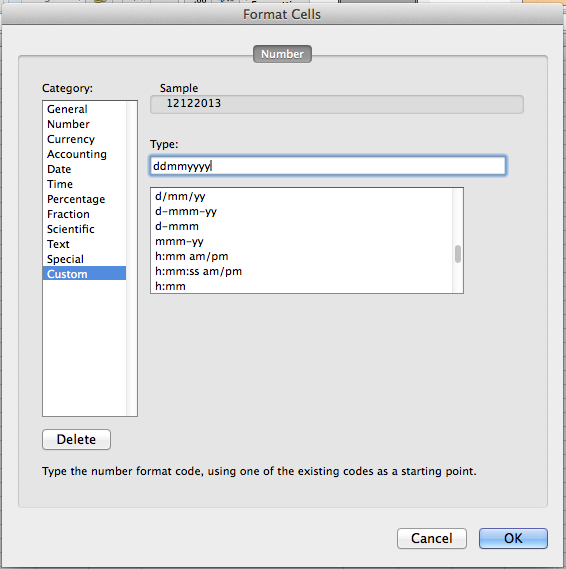This examples shows the date example you gave with a custom format applied:

• dd means show the day in 2 digits (so if the date was the 5th of December, it would appear as 05)
• mm means show the month in 2 digits
• yyyy means show the year in 4 digits.

Note that you could put this combination of dd, mm and yyyy in any order that you want, such as mmddyyyy. Excel will automatically use the underlying date value to show it correctly. You can also use any combination of other characters within the format, as shown in the two options directly below my custom format type in the example above.

Another thing worth noting is that you can use 1 letter, 2 letters, 3 letters or 4 letters. For example:

• Using d will return 12, or 5 on the fifth
• Using dd returns 12 or 05
• Using ddd returns the first three letters of the day (Thu in your example)
• Using dddd returns the full name of the day (Thursday)

This also works with the month value (m, mm, mmm, mmmm). It also works with the year value, but only yy (13) and yyyy (2013) are worth using.

This opens the potential for a date format such as this:

• dddd, dd mmmm, yyyy
• Thursday, 12 December, 2013

The great thing about this is it's only a format, so the date stored in the cell doesn't have to change in any way.

I hope this helps. Let me know if you still have any questions.

Regards

David

### Microsoft Excel

I like the explaining method posted by expert. As I have been studying in Excel study but sometime it is very hard to get idea from the text book but you guys had made easier for us.

### mixed column type

I need to convert a column to short date format. A few of the cells are already in date format, some cells are empty and if I put a 0 in them they convert to the date of 1/0/1900 showing that they are also in date format. But most cells have data looking like 11-15-2012. I've tried highlighting the column and highlighting individual cells and changing the number format, but nothing happens. I've tried the datevalue formula but get #VALUE instead. I've tried option 3 above on cell O9 with 11-15-2012 in it. [=DATE(LEFT(O9,2),MID(O9,4,2),RIGHT(O9,4))] gives me 9/2/1917. I can convert the text in cell O9 to a date if I double click in the cell, but I've got over 14,000 rows and I refuse to double click in all of them. Can you help me?

### I thought maybe I had the

I thought maybe I had the equation wrong so I tried changing the equation to =DATE(RIGHT(O9,4),LEFT(O9,2),MID(O9, 3,2)) That gives me 10/30/2012. (How it got that out of 11-15-2012 I have no idea!)

### Try the DATEVALUE function instead of DATE

Hi Beth

I can reproduce the scenarios you've described but I can't explain them. The only thought that crossed my mind is that your example is in US date formats, whilst I'm using Excel with British date formats set on my computer, but that doesn't seem to fit with the results we're both getting.

However, I think I can solve the problem for you. Try this:

=DATEVALUE(LEFT(O9,2)&"/"&MID(O9,4,2)&"/"&RIGHT(O9,4))

The DATEVALUE function takes a text value and converts it to a date, provided the text string is presented as a valid date.

The formula I've supplied takes each date component from the value in O9 and concatenates them into a string where each date component is separated by a /

As I noted above, the date in O9 (11-15-2012), is in US date format (mm-dd-yyyy) whereas I'm using dd-mm-yy. So the formula given above doesn't work for me - I have to swap the day and month components around. My version of that formula looks like this:

=DATEVALUE(MID(O9,4,2)"/"&LEFT(O9,2)&"/"&RIGHT(O9,4))

I hope that helps - let me know how you get on!

David

### Convert text into number of months

Hi

I'm having trouble converting a series of cells that have time periods such as "1 year 8 months" or "3 months 2 weeks" into the number of months that contains. Can you help? Thanks

### Convert Text to Date Issue

Hello! I hate to do this, but I read your document and comments section, which is very informative, but cannot seem to come up witha solution to my problem. I think my situation is unique, but not impossible. Who knows.